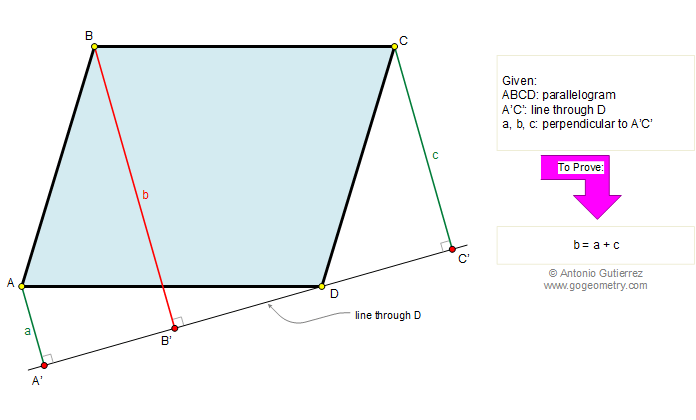Problem 232. Parallelogram, Line through a vertex, Perpendicular lines

The figure shows a parallelogram ABCD, A'C' is a line through the point D. If a, b, and c are the perpendiculars drawn from A, B, and C to the line A'C', respectively, prove that: b = a + c. View or post a solution.